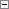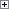Rounds the value of a numeric variable.

NewNumber := ROUND(Number [, Precision] [, Direction])

Parameters

Number

Type: Decimal

The number that you want to round.

Precision

Type: Decimal

This optional parameter determines the precision used when rounding. If you do not specify a Precision parameter, then the following steps are used to specify the precision:

1. The function ReadRounding in Codeunit 1, Application Management, is called. ReadRounding returns a decimal value that is the precision. By default, the ReadRounding function returns the Amount Rounding Precision field from the GLSetup table.
2. If you have customized Codeunit 1 and it does not implement the ReadRounding function, then the precision is specified as 2 digits after the decimal.
Direction

Type: Text or Code

This optional parameter specifies how to round the Number parameter. The default rounding method is '='. The following are the options for rounding:

• '=' rounds up or down to the nearest value (default). Values of 5 or greater are rounded up. Values less than 5 are rounded down.
• '>' rounds up
• '<' rounds downProperty Value/Return Value

Type: Decimal

The rounded result.Example

This example shows how to use the ROUND function. This example requires that you create the following variables and text constant in the C/AL Globals window.

Variable name DataType

DecimalToRound

Decimal

Direction

Text

Precision

Decimal

Result

Decimal

Text Constant ENU Value

Text000

ROUND(%1, %2, %3) returns %4.Copy Code
DecimalToRound := 1234.56789;
Direction := '>';
Precision := 0.001;
Result := ROUND(DecimalToRound, Precision, Direction);
MESSAGE(Text000, Format(DecimalToRound,0,1), Precision, Direction, Result);

On a computer that has the regional format set to English (United States), the message window displays the following:

ROUND(1234.56789, 0.001, >) returns 1,234.568

The following table displays some additional ROUND examples.

Number Precision Direction Rounded number

1234.56789

100

=

1200

1234.56789

0.1

=

1234.6

1234.56789

0.001

=

1234.568

1234.56789

0.001

<

1234.567

1234.56789

0.001

>

1234.568

-1234.56789

100

=

-1200

-1234.56789

0.1

=

-1234.6

-1234.56789

0.001

=

-1234.568

-1234.56789

0.001

<

-1234.567

-1234.56789

0.001

>

-1234.568

When you round down ('<') a negative number, such as -1234.56789, it is rounded down to -1234.567. However, -1234.567 is a mathematically greater value than -1234.56789.

When you round up ('>') a negative number, such as -1234.56789, it is rounded up to -1234.568. However, -1234.568 is a mathematically smaller value than -1234.56789.